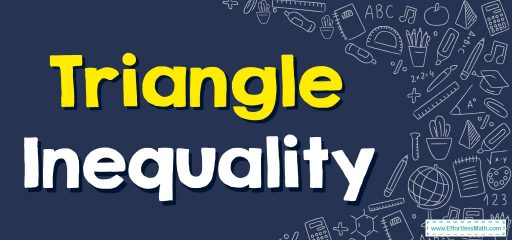# Triangle Inequality

The triangle inequality states that for any triangle, the length of any one side of the triangle must be less than the sum of the lengths of the other two sides.In other words, if you have a triangle with sides a, b, and c, then a + b > c, b + c > a, and c + a > b.

## A step-by-step guide to Using Triangle Inequality Rules

For example, if you have a triangle with sides of lengths 4, 5, and 7, you can check whether it satisfies the triangle inequality:

• 4 + 5 > 7 (true)
• 5 + 7 > 4 (true)
• 7 + 4 > 5 (true)

Since all three conditions are true, this triangle satisfies the triangle inequality and is a valid triangle.

On the other hand, if you have sides of lengths 2, 5, and 10:

• 2 + 5 > 10 (false)
• 5 + 10 > 2 (true)
• 10 + 2 > 5 (true)

In this case, the first condition is false, which means that these side lengths do not form a valid triangle.

### Triangle Inequality – Example 1

Do the given sides form a triangle? $$a=3, b=5, c=8$$
Solution:
Add the first and second lengths. $$3+5=8$$
8 is equal to the third length. So, it is not a triangle.

### Triangle Inequality – Example 2

Do the given sides form a triangle? $$a=7, b=6, c=12$$
Solution:
Add the first and second lengths. $$7+6=13$$
13 is larger than the third length (12). So, they form a triangle.

### What people say about "Triangle Inequality - Effortless Math: We Help Students Learn to LOVE Mathematics"?

No one replied yet.

X
30% OFF

Limited time only!

Save Over 30%

SAVE $5 It was$16.99 now it is \$11.99